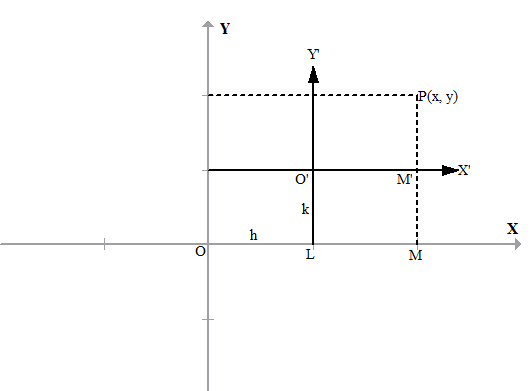Request a call back

# CBSE Class 11-science Answered

<div>Family of lines and shifting of the origin is there in the cbse curriculum..If u will see at the back of the class 11 maths book. u will find the topic under the supplementary material and these topics are taught in schools as well..so i would like u to pls look over these topics as these topics &nbsp;are there in my final exams syllabus and i need notes as well as questions on it..thnk u</div> <div>&nbsp;</div>
Asked by Aditi Srivastava | 08 Dec, 2014, 08:57: PMExpert Answer

Shifting of Origin

In coordinate geometry points are placed in a grid. There are two axes, the horizontal one is x - axis and the vertical one is y - axis. Both the axes intersect at the origin.

On x-axis, positive values of x are placed on the right direction and negative values of x are placed on the left direction from the origin.

Similarly, On y-axis, positive values of y are placed on the up direction and negative values of y are placed on the down direction from the origin.

Some times while solving specific problems, it is easy to solve with a different set of axes.

The original system of axes may be shifted parallel to get the new system of axes or the original axes may be rotated through an angle without changing the origin or a combination of both the processes.  This process is called transformation or change of axes. Hence, it becomes necessary to find the co-ordinates of the point with respect to new coordinate system.

By shifting of origin and coordinate transformation formulas, co-ordinates of a point with respect to original co-ordinate system can be converted to new set of axes.

Now consider the translation of axes. In this transformation, new set of axes are transformed parallel to the original axes and origin is shifted to a new point.

Under the translation process, the coordinates of each point of the plane changed with respect to new set of axes.

Let us consider a point P(x,y) with respect to the axes OX and OY. Therefore, OM = x and MP = y

Let O'(h,k) be the point with respect to original system of axes. Thus, OL = h and O'L=k

Let O'X' and O'Y' be the new set of axes parallel to OX and OY respectively. Thus, O'M' = x' and
M'P = y' are the abscissa and ordinates of a point with respect to new set of axes O'X' and O'Y'

Consider the following figure.From the figure, it is clear that,

OM = OL + LMx = h + x'

MP = MM' + M'Py = k + y'

These are the transformation formulae to find the new set of coordinates.

Family of Lines

The family of straight lines through the intersection of linesThe equation L1 + KL2 = 0 represents a line passing through the intersection of the lines
L1 = 0 and L2 = 0 which is a fixed point. Hence L1 + KL2 = 0 represents a family of straight lines for different values of K, which pass through a fixed point.

Using any one of the following conditions, we can determine the value of K:

1. Given system of lines pass through a point P(x1, y1)

2. Given system of lines is parallel to line L=0

3. Given system of lines is perpendicular to the line L = 0

4. Given system of lines has equal intercepts on the co-ordinate axes.

Answered by Vimala Ramamurthy | 10 Dec, 2014, 05:03: PM

## Application Videos

CBSE 11-science - Maths
Asked by bhunias174 | 30 Mar, 2022, 09:45: PMANSWERED BY EXPERT
CBSE 11-science - Maths
Asked by ankitakhokhar56 | 06 Dec, 2019, 02:51: PMANSWERED BY EXPERT
CBSE 11-science - Maths
Asked by manish.kkd4 | 20 Feb, 2019, 12:20: PMANSWERED BY EXPERT
CBSE 11-science - Maths
Asked by Topperlearning User | 04 Jun, 2014, 01:23: PMANSWERED BY EXPERT
CBSE 11-science - Maths
Asked by Topperlearning User | 26 Sep, 2014, 09:43: AMANSWERED BY EXPERT
CBSE 11-science - Maths
Asked by Topperlearning User | 04 Jun, 2014, 01:23: PMANSWERED BY EXPERT
CBSE 11-science - Maths
Asked by Topperlearning User | 26 Sep, 2014, 01:57: PMANSWERED BY EXPERT
CBSE 11-science - Maths
Asked by Topperlearning User | 04 Jun, 2014, 01:23: PMANSWERED BY EXPERT
CBSE 11-science - Maths
Asked by Topperlearning User | 04 Jun, 2014, 01:23: PMANSWERED BY EXPERT
CBSE 11-science - Maths
Asked by Topperlearning User | 04 Jun, 2014, 01:23: PMANSWERED BY EXPERT